# Circle - 8th grade (13y) - math problems

#### Number of problems found: 292

• Square and circle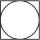Into square is inscribed circle with diameter 10 cm.What is difference between circumference square and circle?
• Circumscription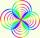Calculate radius of the Circumscribed circle in the rectangle with sides 20 and 19. It can be rectangle inscribed by circle?
• Circle chordWhat is the length x of the chord circle of diameter 115 m, if the distance from the center circle is 11 m?
• Circle r,DCalculate the diameter and radius of the circle if it has length 26.59 cm.
• Circular flowerbed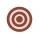Circular flowerbed with diameter 8 m we split by concentric circle to circle and annulus with the same area. Find the radius of the circle.
• Circle - simpleCalculate the area of a circle in dm2, if its circumference is 31.4 cm.
• Desribed circle to rectangle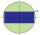Rectangle with sides 6 cm and 4 cm was circumscribed circle. What part of the content of the circle determined by the circumscribed circle occupies a rectangle? Express in perctentages(%).
• Two annulusesThe area of the annular circle formed by two circles with a common center is 100 cm2. The radius of the outer circle is equal to twice the radius of the inner circle. Determine the outside circle radius in centimeters.
• Chord MNChord MN of circle has distance from the center circle S 120 cm. Angle MSN is 64°. Determine the radius of the circle.
• Circle and rectangleA rectangle with sides of 11.7 cm and 175 mm is described by circle. What is its length? Calculate the content area of the circle described by this circle.
• Hexagon in circleCalculate the radius of a circle whose length is 10 cm greater than the circumference of a regular hexagon inscribed in this circle.
• Squaring the Circle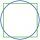Calculating side of the square with the same area as the circle of radius 18.
• Circle's chordsIn the circle there are two chord length 30 and 34 cm. The shorter one is from the center twice than longer chord. Determine the radius of the circle.
• Concentric circlesThere is given a circle K with a radius r = 8 cm. How large must a radius have a smaller concentric circle that divides the circle K into two parts with the same area?
• Rectangle and circle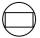The rectangle ABCD has side lengths a = 40 mm and b = 30 mm and is circumscribed by a circle k. Calculate approximately how many cm is circle long.
• Circle in rhombus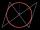In the rhombus is an inscribed circle. Contact points of touch divide the sides to parts of length 19 cm and 6 cm. Calculate the circle area.
• Circle chordCalculate the length of the chord of the circle with radius r = 10 cm, length of which is equal to the distance from the center of the circle.
• OK circleCalculate the radius (circumradius) of the circle described right triangle with hypotenuse long 33 and one cathetus long 17.
• Inscribed circle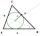The circle inscribed in a triangle has a radius 3 cm. Express the area of the triangle using a, b, c.
• Perimeter of circleCalculate the circumference of described circle to the triangle with sides 9,12,15 cm.

Do you have an interesting mathematical word problem that you can't solve it? Submit a math problem, and we can try to solve it.

We will send a solution to your e-mail address. Solved examples are also published here. Please enter the e-mail correctly and check whether you don't have a full mailbox.

Please do not submit problems from current active competitions such as Mathematical Olympiad, correspondence seminars etc...